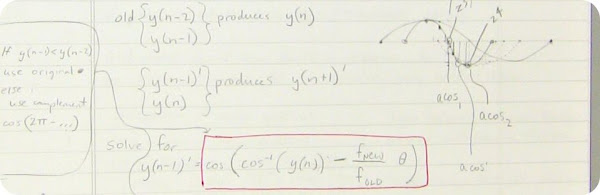# A Precise Digital OscillatorThe continuous phase precision oscillator is a C++ class for use in VST plugins. It generates sinusoids with high efficiency using only one multiply per sample.

It is a modification of the digital sinusoidal oscillator represented by the following difference equation, where$\omega$ is the desired frequency of oscillation:$y_{n+1} = 2\: cos\: \omega\: y_{n} - y_{n-1}$

This difference equation predicts that when the frequency is changed by$\lambda$ while the oscillator is running, the amplitude will deviate. We can overcome this problem by simultaneously recalculating the memory values$y_{n}$ and$y_{n-1}$ whenever we wish to change the oscillator frequency.

The solution for the new memory value is calculated whenever frequency is updated:$y_{n-1}' = \cos \left [\arccos(y_n)-\frac{\omega'}{\omega}\:\theta\right ]$

Audio sample of the oscillator as frequency modulator:

COsc.cpp
COsc.h

## References

 J. W. Gordon and J. O. Smith, “A sine generation algorithm for VLSI applications,'' in Proceedings of the 1985 International Computer Music Conference, Vancouver, Computer Music Association, 1985

 J.O. Smith and P.R. Cook, “The Second-Order Digital Waveguide Oscillator”, Proceedings of the 1992 International Computer Music Conference, San Jose, 1992.

 R. Andraka, “A survey of CORDIC algorithms for FPGA based computers”, Proceedings of the 1998 ACM/SIGDA sixth international symposium on Field programmable gate arrays, Monterey, CA., 1998.

 P. A. Regalia, S. K. Mitra, and P. P. Vaidyanathan, “The Digital All-Pass Filter: A Versatile Signal Processing Building Block”, Proceedings of the IEEE, vol. 76, No. 1, 1988.

 J. Dattorro. “The Implementation of Recursive Digital Filters for High-Fidelity Audio”, J. Audio Eng. Soc., vol. 36, No. 11, 1988.

1.#### Yann

/  April 18, 2012 Quote

Hi,